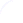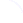Search
 Submission Procedure share: |available in:   PDF (156 kB) PS (254 kB)

get:
 Similar Docs BibTeX Write a comment
get:

DOI:   10.3217/jucs-013-11-1615

### Analysis of two Sweep-line Algorithms for Constructing Spanning Trees and Steiner Trees

Adrian Dumitrescu (University of Wisconsin-Milwaukee, USA)

Csaba D. Tóth (Massachusetts Institute of Technology, USA)

Abstract: We give a tight analysis of an old and popular sweep-line heuristic for constructing a spanning tree of a set of n points in the plane. The algorithm sweeps a vertical line across the input points from left to right, and each point is connected by a straight line segment to the closest point left of (or on) the sweep-line. If W denotes the weight the Euclidean minimum spanning tree (EMST), the spanning tree constructed by the sweep-line algorithm has weight O(W log n), and this bound is asymptotically tight. We then analyze a sweep-line heuristic for constructing a Steiner tree, in which a vertical line is swept across the input points from left to right, and each point is connected by a straight line segment to the closest point on edges or vertices of the current tree (on the left of the sweep line). We show that this algorithm achieves an approximation ratio of O(log n), and describe a class of instances where this ratio is Ω(log n / log log n). Our results give almost complete answers to two old open questions from the 1970s.

Keywords: approximation ratio, heuristic, minimum Steiner tree, minimum spanning tree, sweep-line

Categories: F.2.2, G.2.2